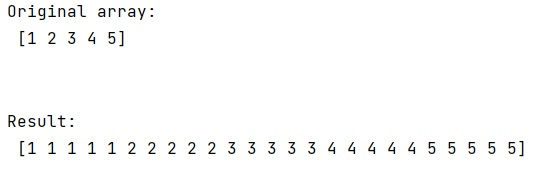# How to repeat each element of a NumPy array 5 times?

Learn, how to repeat each element of a NumPy array 5 times?
Submitted by Pranit Sharma, on March 09, 2023

## Repeating each element of a NumPy array 5 times

Suppose that we are given a numpy array and we need to repeat each of its elements 5 times with the array itself.

For example, if we have an array as [1,2,3,4], we need the output as [1,1,1,1,1,2,2,2,2,2,3,3,3,3,3,4,4,4,4,4].

To repeat each element of a numpy array 5 times, we can use the numpy.repeat() function which is used to repeat the elements of an array N number of times, where N is the parameter, it accepts.

We can also repeat the whole array N number of times. For that purpose, we can use the numpy tile() function.

Let us understand with the help of an example,

## Python code to repeat each element of a NumPy array 5 times

```# Import numpy
import numpy as np

# Creating a numpy array
arr = np.array([1,2,3,4,5])

# Display Original array
print("Original array:\n",arr,"\n\n")

# Repeating each element 5 times
res = np.repeat(arr,5)

# Display result
print("Result:\n",res)
```

### OutputLanguages: » C » C++ » C++ STL » Java » Data Structure » C#.Net » Android » Kotlin » SQL
Web Technologies: » PHP » Python » JavaScript » CSS » Ajax » Node.js » Web programming/HTML
Solved programs: » C » C++ » DS » Java » C#
Aptitude que. & ans.: » C » C++ » Java » DBMS
Interview que. & ans.: » C » Embedded C » Java » SEO » HR
CS Subjects: » CS Basics » O.S. » Networks » DBMS » Embedded Systems » Cloud Computing
» Machine learning » CS Organizations » Linux » DOS
More: » Articles » Puzzles » News/Updates